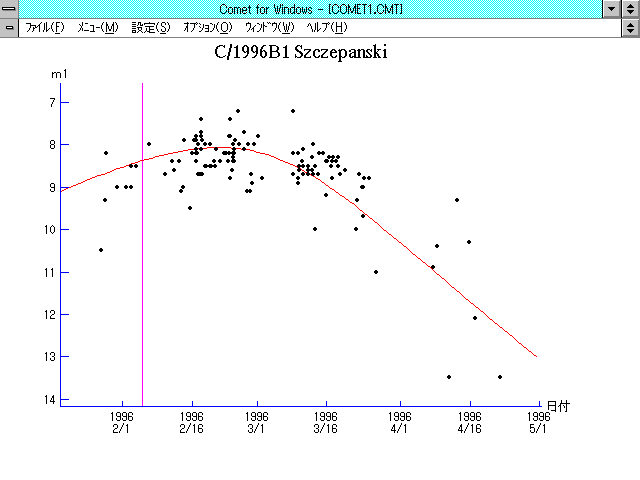# \$B%7%'%Q%s%9%-!

C/1996 B1 ( Szczepanski )###\$B%W%m%U%#!<%k(B

 \$BH/8+F|(B 1996\$BG/(B1\$B7n(B27\$BF|(B \$BH/8+8wEY(B 10.5\$BEy(B \$BH/8+ Edward W. Szczepanski (Houston, TX)

###\$B50F;MWAG(B

```   The following improved orbital elements, by Kenji Muraoka
are from 458 observations  1996 Jan. 28  to  1996 June  8,
perturbations by  9 planets and 5 minor planets were taken
into account.   The mean residual is +/- 0.70 arc seconds.

Epoch  =  1996 Feb.  7.0  TT       JDT = 2450120.5
T  =  1996 Feb.  6.91369       +/- 0.00020 (m.e.) TT
Peri. =  151.28265                +/- 0.00020
Node  =  345.44199                +/- 0.00007   (2000.0)
Incl. =   51.91826                +/- 0.00019
q  =    1.4487789              +/- 0.0000033 AU
e  =    0.9907294              +/- 0.0000149
1/a  =   +0.0063989              +/- 0.0000103 1/AU
orig. 1/a  =   +0.0062184
fut.  1/a  =   +0.0069000
```

###\$B@1?^(B1995\$BG/(B 9\$B7n(B10\$BF|!A(B1996\$BG/(B 3\$B7n(B18\$BF|(B1996\$BG/(B 2\$B7n(B27\$BF|!A(B1996\$BG/(B 8\$B7n(B25\$BF|(B

###\$B8wEYJQ2=(B

```        m1 = 4.2 + 5 log\$B&\$(B + 30.0 log r
```##### \$B50F;MWAG\$OB<2,7r<#;a\$N7W;;\$K\$h\$k\$b\$N\$G\$9!#(B \$B@1?^\$O(B StellaNavigator Ver.2.0 for Windows (\$B%"%9%H%m%"!<%D(B \$BJTCx(B / \$B%"%9%-!<=PHG6I4)(B) \$B\$G:n@.\$7\$?\$b\$N\$G\$9!#(B \$B8wEY%0%i%U\$O(BComet for Windows\$B\$G:n@.\$7\$?\$b\$N\$G\$9!#(B